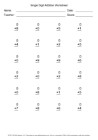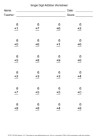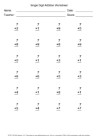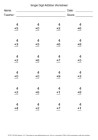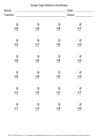# Search

About 506 Search Results Matching Types of Worksheet, Worksheet Section, Generator, Generator Section, Subjects matching Math, Type matching Worksheet, Similar to Reading a Protractor Worksheet 2, Page 2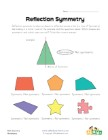## Symmetry Worksheet 2

Which shapes are symmetric and which are not?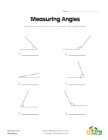## Measuring Angles Worksheet 1

Measure each of the angles with a protractor and w...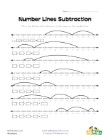## Number Lines Subtraction Worksheet 2

Fill in the blanks in the equations by using the n...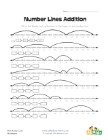## Number Lines Addition Worksheet 2

Fill in the blanks in the equations by using the n...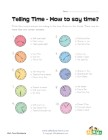## How to Say the Time 2

Circle the correct way to say each of the times sh...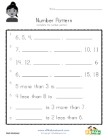## Number Pattern Worksheet 2

Fill in the blanks to complete the number patterns...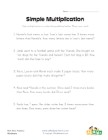## Simple Multiplication Word Problems Worksheet

Use simple multiplication to solve the 5 word prob...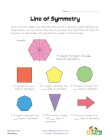## Lines of Symmetry Worksheet 2

Look at the shapes, count and write down how many ...